# Volume of a Rectangular Prism

Filling and wrapping is a great way to think about volume and surface area. When you fill something up, the space that is filled is the volume. When you wrap up a present or cover something you are now working with surface area.

Companies need to know the volume of a box in order to determine how much of their product will fit inside.

Here is an example: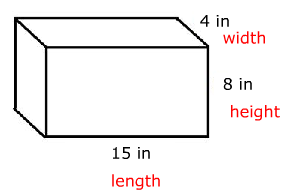The volume is equal to the number of 1 in x 1 in x 1 in cubes we can fit inside this box.

There could be 15 cubes across the length by 4 across the width, making a bottom layer of 15 x 4 = 60 cubes. You can also think of this as the area of the base.

Notice that the height of the box is 8 in. That means that we could fit 8 layers of 60 cubes.In other words, the volume of this box is 480 cubic inches.

This could be simplified to the formulas V = Bh or V = l w h for all rectangular prisms.

Let's Practice! Determine the volume of each prism.

1.)V = l w h

V = (25 in.)(3 in.)(7 in.)

V = (75 in2)(7 in)

V = 525 in3

2.)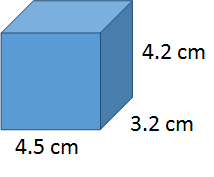V = l w h

V = (4.5 cm)(3.2 cm)(4.2 cm)

V = 60.48 cm3

3.)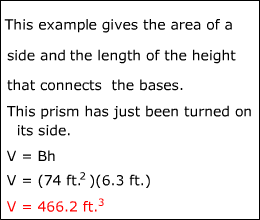4.)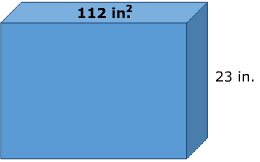V = Bh

V = (112 in.2)(23 in.)

V = 2576 in.3

Let's Review

The volume of a prism is the space that fills it. You can determine the volume of a rectangular prism by either multiplying the area of the base times the height or by multiplying the length, width and height.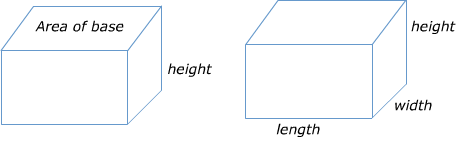Related Links: Math Geometry Area of Rectangular Prism Volume of a Rectangular Prism